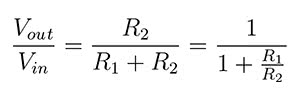# Resistor Divider Calculator And Part Optimizer

## Finding The Right Resistors

Use this tool to find the optimum values for a resistor divider given a few parameters. If you already have a set of resistors to optimize over, use the Custom resistor set below. Discreet search space analyzed by using linear algebra package Numeric.js. Supports a minimum Vout/Vin ratio of 0.1.Vin
Volts
Vout
Volts

#### Resistor Set & Tolerance

##### R1 and R2 results should be multipled by a common unit denomination of your choice. For example, results of R1 = 10 and R2 = 27 can be used as 100 and 270, 10k and 27k, 100k and 270k, etc.

The above optimization works across the resistor divider equation at discreet points defined by the resistor set chosen. The output voltage tolerance is calculated using the provided resistor tolerance, again using the resistor divider equation.You’re welcome ;-). Find out more about the resistor sets mentioned above at this classic site.